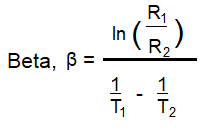﻿ NTC Thermistor Beta Calculator ﻿# NTC Thermistor Beta CalculatorEnter the Resistance, R1: Ω (ohms) KΩ (kilohms) MΩ (megohms) Enter the Temperature, T1: K (kelvin) C (celsius) F (fahrenheit) Enter the Resistor, R2: Ω (ohms) KΩ (kilohms) MΩ (megohms) Enter the Temperature, T2: K (kelvin) C (celsius) F (fahrenheit)

Beta, β:

This NTC thermistor beta calculator calculates the beta (β) value of the NTC thermistor based on two points of resistances of the thermistor with their corresponding temperatures.

So this calculator needs 2 different, distinct resistance values along with their corresponding temperatures to be able to calculate the beta value of the thermistor.

The beta value is an indication of the shape of the curve representing the relationship between resistance and temperature of an NTC thermistor.

Know that the curve of the resistance vs temperature of an NTC thermistor is not linear unless the NTC thermistor is specifically a linear thermistor; otherwise, the relationship is logarithmic and it varies according to the specific type of NTC thermistor being used.

The beta value helps to paint the curve for the specific thermistor in use .

As stated before, this calculator needs 2 pairs of resistance-temperature values, and then it can compute the beta value. To use this calculator, simply enter in resistance R1 with its corresponding temperature value, T1, and resistance R2 with its corresponding temperature value, T2, and then press 'Calculate'. The resultant beta value will then be calculated and displayed.

The beta value is in unit K (or Kelvin).

﻿